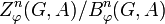# Continuous cohomology group

## Definition

Suppose$G$ and$A$ are topological groups (typically, taken to be T0 topological groups) such that$A$ is an abelian group and there is a topological group action of$G$ on$A$, i.e., a homomorphism$\varphi:G \to \operatorname{Aut}(A)$ such that the induced map$G \times A \to A$ is continuous. We can then define continuous cohomology groups$H^n_\varphi(G;A)$, each of which is an abelian group.

When$\varphi$ is understood from context, the subscript${}_\varphi$ may be omitted in the notation for the cohomology group, as well as the notation for the groups of$n$-cocycles and$n$-coboundaries.

### Definition in terms of homological algebra

Note that these definitions are the same as those for the cohomology group in the discrete setting, with the following important difference: the$\mathbb{Z}G$-modules here are abelian topological groups with topological group actions of$G$ on them, and the morphisms between them must be continuous in addition to being equivariant under the$G$-action. Also, for the bar resolution, the cochains in the cochain complex comprise only the continuous functions.

No. Shorthand Detailed description of$H^n_\varphi(G,A)$, the$n^{th}$ cohomology group
1 Complex based on arbitrary projective resolution Let$\mathcal{F}$ be a projective resolution for$\mathbb{Z}$ as a$\mathbb{Z}G$-module with the trivial action. Let$\mathcal{C}$ be the complex$\operatorname{Hom}_{\mathbb{Z}G}(\mathcal{F},A)$. The cohomology group$H^n_\varphi(G,A)$ is defined as the$n^{th}$ cohomology group for this complex.
2 Complex based on arbitrary injective resolution (works if category of$\mathbb{Z}G$-modules has enough injectives!) Let$\mathcal{I}$ be an injective resolution for$A$ as a$\mathbb{Z}G$-module with the specified action$\varphi$. Let$\mathcal{D}$ be the complex$\operatorname{Hom}_{\mathbb{Z}G}(\mathbb{Z},\mathcal{I})$ where$\mathbb{Z}$ has the structure of a trivial action$\mathbb{Z}G$-module. The cohomology group$H^n_\varphi(G,A)$ is defined as the$n^{th}$ cohomology group for this complex.
3 As an$\operatorname{Ext}$ functor$\operatorname{Ext}^n_{\mathbb{Z}G}(\mathbb{Z},A)$ where$\mathbb{Z}$ is a trivial$\mathbb{Z}G$-module and$A$ has the module structure specified by$\varphi$.
4 As a right derived functor$H^n_\varphi(G,A) = R^n(-^G)(A)$, i.e., it is the$n^{th}$ right derived functor of the invariants functor for$G$ (denoted$-^G$) evaluated at$A$. The invariants functor sends a$\mathbb{Z}G$-module to its submodule of elements fixed by all elements of$G$.
5 Explicit, using the bar resolution$H^n_\varphi(G,A)$, is defined as the quotient$Z^n_\varphi(G,A)/B^n_\varphi(G,A)$ where$Z^n_\varphi(G,A)$ is the group of cocycles for the action and$B^n_\varphi(G,A)$ is the group of coboundaries.
5' Explicit, using the normalized bar resolution Same as definition (5), but we use normalized cocycles and normalized coboundaries instead of arbitrary cocycles and coboundaries.